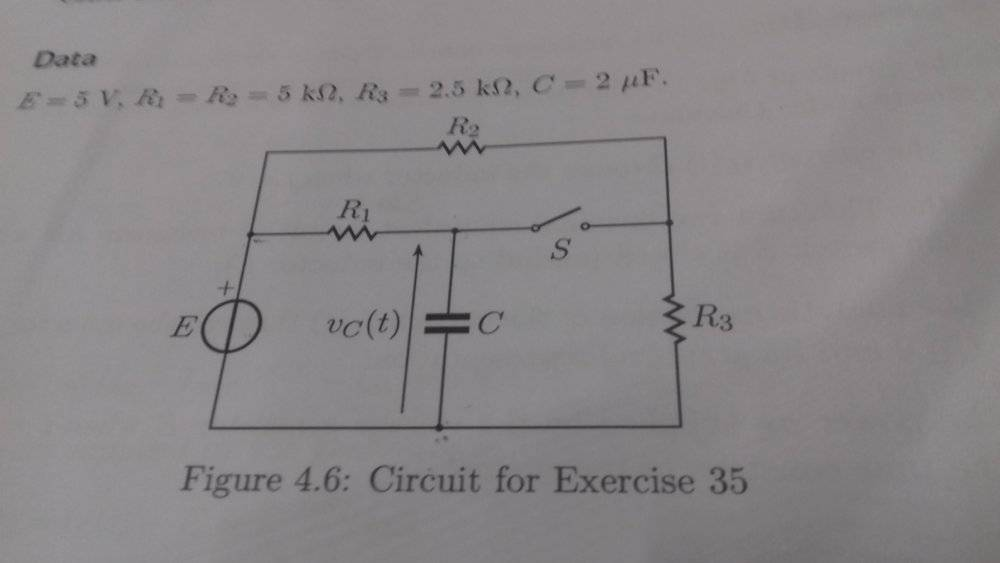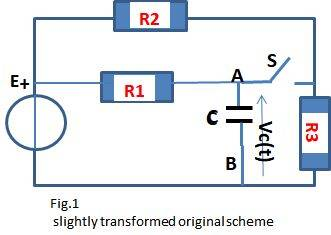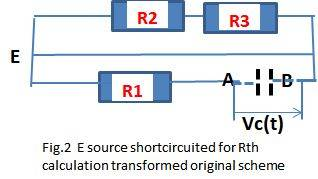# Problem with the Thevenin equivalent resistance

• Engineering
Amaelle
Homework Statement:
Find the thevenin equivalent resistance from the capacitor point of view
Relevant Equations:
no equation needed just the diagram
Good day
I have a problem to find the equivalent resistance from the capacitor point of viewthe solution says it's R1 but for me we still have R2 and R3 we have not deal with !

Staff Emeritus
If that's your attempt at solution, you didn't try very hard.

The schematic shows an open switch S. If the switch was closed, the equivalent would be different. So how would you make the equivalent with the switch open?

Amaelle
the question is with switch open :)

Mentor
What is the usual procedure for finding the Thevenin resistance?•